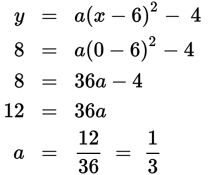# SAT Math Multiple Choice Question 283: Answer and Explanation

### Test Information

Question: 283

13.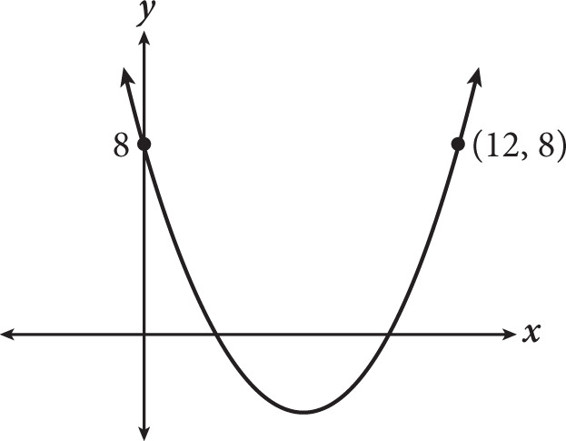The range of the parabola shown in the graph is y ≥ -4. If the equation y = ax2 + bx + c is used to represent the graph, what is the value of a?

• A.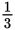• B.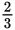• C.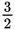• D. 3

Explanation:

A

Difficulty: Hard

Getting to the Answer: The vertex of the parabola shown must lie on its axis of symmetry, which is halfway between the two points (0, 8) and (12, 8). This means the x-coordinate of the vertex is halfway between 0 and 12, which is 6. To find the y-coordinate of the vertex, look at the range: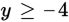means that the minimum value of the graph, and hence the y-coordinate of the vertex, is -4. Now, use the vertex (6, -4) to set up a quadratic equation in vertex form: y = a(x - h)2 + k. The result is y = a(x - 6)2 - 4. Plug in either of the given points for x and y to find the value of a. Using (0, 8), the result is: